Example Questions

← Previous 1 3 4

Example Question #6 : How To Find The Length Of The Diagonal Of A Square

The perimeter of a square is 48. What is the length of its diagonal?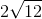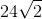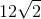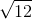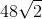Explanation:

Perimeter = side * 4

48 = side * 4

Side = 12

We can break up the square into two equal right triangles. The diagonal of the sqaure is then the hypotenuse of these two triangles.

Therefore, we can use the Pythagorean Theorem to solve for the diagonal: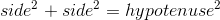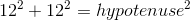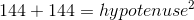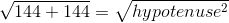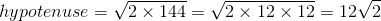Example Question #1 : How To Find The Length Of The Diagonal Of A Square

What is the area of a square with a diagonal of length? Round to the nearest hundredth.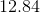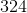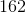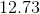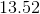Explanation:

Based on the data provided, the square could be drawn like this: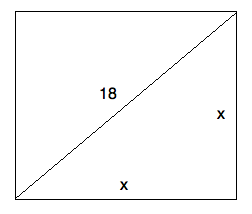Based on this, you can use the Pythagorean theorem to find: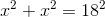or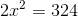From this, you know that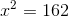Since the area is equal to, this is your answer!

Example Question #7 : How To Find The Length Of The Diagonal Of A Square

The perimeter of a square isunits. How many units long is the diagonal of the square?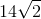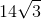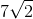Explanation:

From the perimeter, we can find the length of each side of the square. The side lengths of a square are equal by definition therefore, the perimeter can be rewritten as,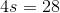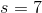Then we use the Pythagorean Theorme to find the diagonal, which is the hypotenuse of a right triangle with each leg being a side of the square.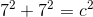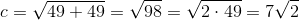Example Question #2 : How To Find The Length Of The Diagonal Of A Square

Find the diagonal of a square with side length.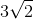Explanation:

To solve, simply realize the triangle that is made bysides and the diagonal is an isoceles right triangle. Thus, the hypotenuse is side length time square root of. Thus,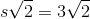Example Question #3 : How To Find The Length Of The Diagonal Of A Square

Find the length of a diagonal of a square whose side length is.Explanation:

To solve, simply remember that the diagonal forms an isoceles right triangle. Thus, the diagonal is: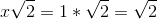Example Question #8 : How To Find The Area Of A Square

How much more area does a square with a side of 2r have than a circle with a radius r?  Approximate  π by using 22/7.

1/7 square units

12/14 square units

4/7 square units

6/7 square units

6/7 square units

Explanation:

The area of a circle is given by A = πr2 or 22/7r2

The area of a square is given by A = s2 or (2r)2 = 4r2

Then subtract the area of the circle from the area of the square and get 6/7 square units.

Example Question #9 : How To Find The Area Of A Square

If the perimeter of a square is 44 centimeters, what is the area of the square in square centimeters?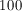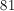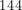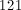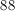Explanation:

Since the square's perimeter is 44, then each side is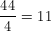.

Then in order to find the area, use the definition that the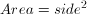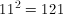Example Question #10 : How To Find The Area Of A Square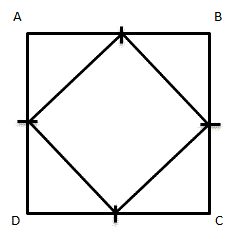Given square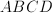, with midpoints on each side connected to form a new, smaller square.  How many times bigger is the area of the larger square than the smaller square?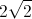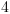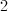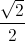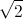Explanation:

Assume that the length of each midpoint is 1.  This means that the length of each side of the large square is 2, so the area of the larger square is 4 square units.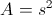To find the area of the smaller square, first find the length of each side.  Because the length of each midpoint is 1, each side of the smaller square is(use either the Pythagorean Theorem or notice that these right trianges are isoceles right trianges, so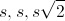can be used).

The area then of the smaller square is 2 square units.

Comparing the area of the two squares, the larger square is 2 times larger than the smaller square.

Example Question #11 : How To Find The Area Of A Square

If a completely fenced-in square-shaped yard requires 140 feet of fence, what is the area, in square feet, of the lot?

1225

140

4900

70

35

1225

Explanation:

Since the yard is square in shape, we can divide the perimeter(140ft) by 4, giving us 35ft for each side. We then square 35 to give us the area, 1225 feet.

Example Question #1 : How To Find The Area Of A Square

The perimeter of a square is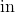. What is its area?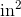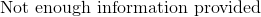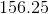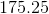Explanation:

The perimeter of a square is very easy to calculate. Since all of the sides are the same in length, it is merely: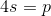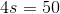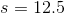From this, you can calculate the area merely by squaring the side's value: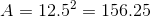← Previous 1 3 4